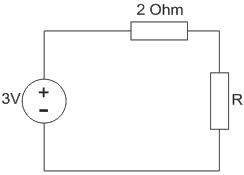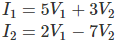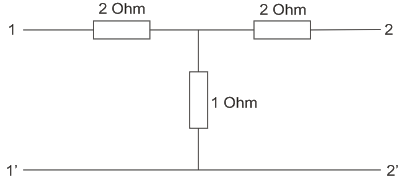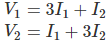# MCQs on Electric Circuits

##### Page 52 of 63. Go to page 1 2 3 4 5 6 7 8 9 10 11 12 13 14 15 16 17 18 19 20 21 22 23 24 25 26 27 28 29 30 31 32 33 34 35 36 37 38 39 40 41 42 43 44 45 46 47 48 49 50 51 52 53 54 55 56 57 58 59 60 61 62 63
01․ Two resistors R1 and R2 give combined resistance of 4.5 Ohm when connected in series and 1 Ohm when in parallel, the resistances are
2.2 Ohm and 2.3 Ohm.
1.5 Ohm and 3 Ohm.
2 Ohm and 2.5 Ohm.
1.25 Ohm and 3.25 Ohm.

R1 + R2 = 4.5; R1R2/ (R1 + R2) = 1; R1R2 = 4.5; Solving R1 = 3 and R2 = 1.5 .

02․ Three resistors each R Ohm is connected to form a triangle. The equivalent resistance across any terminals is
2R/3.
3R/2.
R/2.
R.

R || (R+R)

03․ How many 200W / 220V bulbs connected in series across 220V supply will consume 100W?
1.
2.
4.
Not possible.

When ‘n’ number of bulbs are connected in series, voltage across each lamp will be 220/n V as they are of same ratings. If voltage is reduced n times, the power consumed reduces by n2 times. So, n×P/n2 = 100W. P = 200W. Therefore n = 2.

04․ The terminals across the source are ___________ if a current source is to be neglected.
short circuited.
open circuited.
replaced by a shunt resistance.
replaced by a series resistance.

If a circuit is open no current will flow in the circuit.

05․ The terminals across the source are ___________ if a voltage source is to be neglected.
open circuited.
short circuited.
replaced by a shunt resistance.
replaced by a series resistance.

There will be no voltage drop across the terminals if they are short circuited.

06․ A load is connected to an active network. At the terminals to which the load is connected, RTH = 10 Ohm, VTH = 60V. Then maximum power supplied to the load is
90W.
60W.
180W.
360W.

Maximum power transferred is given by VTH2/4RTH.

07․ The maximum power that can be transferred to the load resistor R from the voltage source in the figure is6/8W.
9/8 W.
7.5 W.
1 W.

Maximum power is transferred when R = 2 Ohm and the value of maximum power is 32/(4×2) = 9/8W.

08․ A two port network is defined by the relationThe value of Z12 is
3/41.
-3.
3.
2/31.

Y12 = 3, ΔY = -41; therefore, Z21 = -Y12/ΔY.

09․ Find the Z parameters of the circuit shown in figure.Z11 = 2; Z12 = 1; Z21 = 1; Z22 = 2
Z11 = 3; Z12 = 1; Z21 = 1; Z22 = 3
Z11 = 3; Z12 = 3; Z21 = 3; Z22 = 3
Z11 = 1; Z12 = 1; Z21 = 1; Z22 = 1

Applying KVL in the left hand side loop,10․ Condition for reciprocity in case of ABCD parameters is